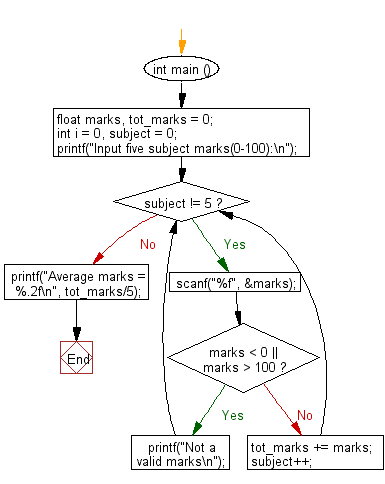﻿ C : Calculate the average of five subject marks of a student

# C Exercises: Read five subject marks (0-100) of a student and calculate the average

## C Basic Declarations and Expressions: Exercise-118 with Solution

Write a C program that reads five subject marks (0-100) of a student and calculates the average of these marks.

Sample Solution:

C Code:

``````#include <stdio.h>

int main () {
float marks, tot_marks = 0;
int i = 0, subject = 0;

// Prompt user for input
printf("Input five subject marks(0-100):\n");

// Loop to read marks for five subjects
while (subject != 5) {

// Read a float value 'marks' from user
scanf("%f", &marks);

// Check if 'marks' is within valid range (0-100)
if (marks < 0 || marks > 100) {
printf("Not a valid marks\n"); // Print an error message
}
else {
tot_marks += marks; // Accumulate valid marks
subject++; // Increment subject count
}
}

// Calculate and print the average marks
printf("Average marks = %.2f\n", tot_marks/5);

return 0; // End of program
}
``````

Sample Output:

```Input five subject marks(0-100):
75
84
56
98
68
Average marks = 76.20
```

Flowchart:C programming Code Editor:

What is the difficulty level of this exercise?

Test your Programming skills with w3resource's quiz.

﻿